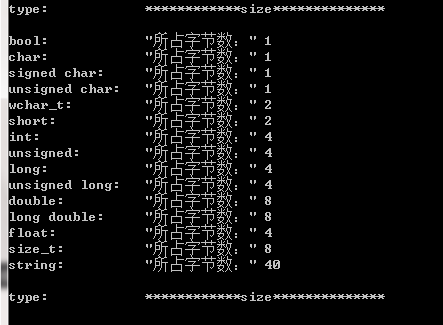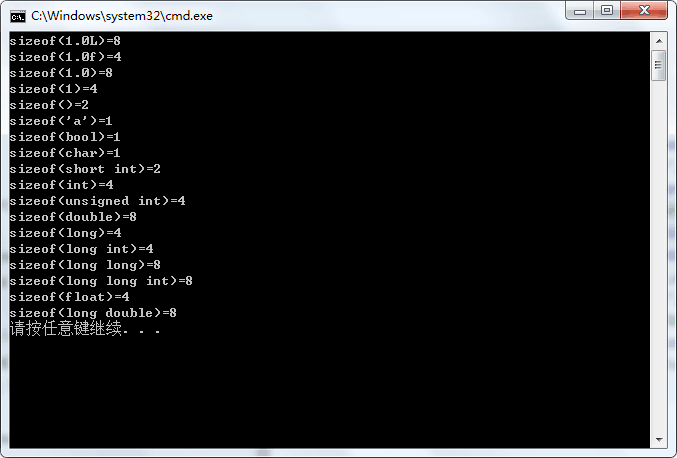• 各种数据类型所占字节
千次阅读
2021-11-11 14:50:39

Java基本数据类型及所占字节大小
一、Java基本数据类型
基本数据类型有8种：byte、short、int、long、float、double、boolean、char

分为4类：整数型、浮点型、布尔型、字符型。

整数型：byte、short、int、long

浮点型：float、double

布尔型：boolean

字符型：char

二、各数据类型所占字节大小
1个字节8位
计算机的基本单位：bit .　　一个bit代表一个0或1

byte：1byte = 8bit　　　　 1个字节是8个bit

short：2byte

int：4byte

long：8byte

float：4byte

double：8byte

boolean：1byte

char：2bytejava 开发语言 后端
更多相关内容
• void DataLength() { qDebug() << “type: \t\t” << “size**” << endl; qDebug() <... QString::fromLocal8Bit(“所占字节数：”) <... QString::fromLocal8Bit(“所占字节数：”) <
void DataLength()
{
qDebug() << "type: \t\t" << "************size**************" << endl;
qDebug() << "bool: \t\t" << QString::fromLocal8Bit("所占字节数：") << sizeof(bool);
qDebug() << "char: \t\t" << QString::fromLocal8Bit("所占字节数：") << sizeof(char);
qDebug()  << "signed char: \t" << QString::fromLocal8Bit("所占字节数：") << sizeof(signed char);
qDebug()  << "unsigned char: \t" << QString::fromLocal8Bit("所占字节数：") << sizeof(unsigned char);
qDebug()  << "wchar_t: \t" << QString::fromLocal8Bit("所占字节数：" )<< sizeof(wchar_t);
qDebug()  << "short: \t\t" << QString::fromLocal8Bit("所占字节数：") << sizeof(short);
qDebug()  << "int: \t\t" << QString::fromLocal8Bit("所占字节数：") << sizeof(int);
qDebug()  << "unsigned: \t" <<QString::fromLocal8Bit( "所占字节数：") << sizeof(unsigned);
qDebug()  << "long: \t\t" << QString::fromLocal8Bit("所占字节数：" )<< sizeof(long);
qDebug()  << "unsigned long: \t" <<QString::fromLocal8Bit( "所占字节数：" )<< sizeof(unsigned long);
qDebug()  << "double: \t" << QString::fromLocal8Bit("所占字节数：") << sizeof(double);
qDebug()  << "long double: \t" << QString::fromLocal8Bit("所占字节数：") << sizeof(long double);
qDebug()  << "float: \t\t" << QString::fromLocal8Bit("所占字节数：" )<< sizeof(float);
qDebug()  << "size_t: \t" << QString::fromLocal8Bit("所占字节数：") << sizeof(size_t);
qDebug()  << "string: \t" << QString::fromLocal8Bit("所占字节数：") << sizeof(string) << endl;
qDebug()  << "type: \t\t" << "************size**************" << endl;
}cout << "type: \t\t" << "************size**************" << endl;
cout << "bool: \t\t" << "所占字节数：" << sizeof(bool);
cout << "\t最大值：" << (numeric_limits<bool>::max)();
cout << "\t\t最小值：" << (numeric_limits<bool>::min)() << endl;
cout << "char: \t\t" << "所占字节数：" << sizeof(char);
cout << "\t最大值：" << (numeric_limits<char>::max)();
cout << "\t\t最小值：" << (numeric_limits<char>::min)() << endl;
cout << "signed char: \t" << "所占字节数：" << sizeof(signed char);
cout << "\t最大值：" << (numeric_limits<signed char>::max)();
cout << "\t\t最小值：" << (numeric_limits<signed char>::min)() << endl;
cout << "unsigned char: \t" << "所占字节数：" << sizeof(unsigned char);
cout << "\t最大值：" << (numeric_limits<unsigned char>::max)();
cout << "\t\t最小值：" << (numeric_limits<unsigned char>::min)() << endl;
cout << "wchar_t: \t" << "所占字节数：" << sizeof(wchar_t);
cout << "\t最大值：" << (numeric_limits<wchar_t>::max)();
cout << "\t\t最小值：" << (numeric_limits<wchar_t>::min)() << endl;
cout << "short: \t\t" << "所占字节数：" << sizeof(short);
cout << "\t最大值：" << (numeric_limits<short>::max)();
cout << "\t\t最小值：" << (numeric_limits<short>::min)() << endl;
cout << "int: \t\t" << "所占字节数：" << sizeof(int);
cout << "\t最大值：" << (numeric_limits<int>::max)();
cout << "\t最小值：" << (numeric_limits<int>::min)() << endl;
cout << "unsigned: \t" << "所占字节数：" << sizeof(unsigned);
cout << "\t最大值：" << (numeric_limits<unsigned>::max)();
cout << "\t最小值：" << (numeric_limits<unsigned>::min)() << endl;
cout << "long: \t\t" << "所占字节数：" << sizeof(long);
cout << "\t最大值：" << (numeric_limits<long>::max)();
cout << "\t最小值：" << (numeric_limits<long>::min)() << endl;
cout << "unsigned long: \t" << "所占字节数：" << sizeof(unsigned long);
cout << "\t最大值：" << (numeric_limits<unsigned long>::max)();
cout << "\t最小值：" << (numeric_limits<unsigned long>::min)() << endl;
cout << "double: \t" << "所占字节数：" << sizeof(double);
cout << "\t最大值：" << (numeric_limits<double>::max)();
cout << "\t最小值：" << (numeric_limits<double>::min)() << endl;
cout << "long double: \t" << "所占字节数：" << sizeof(long double);
cout << "\t最大值：" << (numeric_limits<long double>::max)();
cout << "\t最小值：" << (numeric_limits<long double>::min)() << endl;
cout << "float: \t\t" << "所占字节数：" << sizeof(float);
cout << "\t最大值：" << (numeric_limits<float>::max)();
cout << "\t最小值：" << (numeric_limits<float>::min)() << endl;
cout << "size_t: \t" << "所占字节数：" << sizeof(size_t);
cout << "\t最大值：" << (numeric_limits<size_t>::max)();
cout << "\t最小值：" << (numeric_limits<size_t>::min)() << endl;
cout << "string: \t" << "所占字节数：" << sizeof(string) << endl;
// << "\t最大值：" << (numeric_limits<string>::max)() << "\t最小值：" << (numeric_limits<string>::min)() << endl;
cout << "type: \t\t" << "************size**************" << endl;
system("pause");

展开全文• #include&amp;lt;stdio.h&amp;gt; void main() { printf(&quot;sizeof(1.0L)=%d\n&quot;,sizeof(1.0L));//8 long double printf(&quot;sizeof(1.0f)=%d\n&quot;,sizeof(1.0f));...,si
#include<stdio.h>
void main()
{
printf("sizeof(1.0L)=%d\n",sizeof(1.0L));//8 long double
printf("sizeof(1.0f)=%d\n",sizeof(1.0f));//4 float
printf("sizeof(1.0)=%d\n",sizeof(1.0));//8 double
printf("sizeof(1)=%d\n",sizeof(1));//4 int
printf("sizeof(" ")=%d\n",sizeof(" "));//2
printf("sizeof('a')=%d\n",sizeof('a'));//2
printf("sizeof(bool)=%d\n",sizeof(bool));//1
printf("sizeof(char)=%d\n",sizeof(char));//1
printf("sizeof(short int)=%d\n",sizeof(short int));//2
printf("sizeof(int)=%d\n",sizeof(int));//4
printf("sizeof(unsigned int)=%d\n",sizeof(unsigned int));//4
printf("sizeof(double)=%d\n",sizeof(double));//8
printf("sizeof(long)=%d\n",sizeof(long));//4
printf("sizeof(long int)=%d\n",sizeof(long int));//4
printf("sizeof(long long)=%d\n",sizeof(long long));//8
printf("sizeof(long long int)=%d\n",sizeof(long long int));//8
printf("sizeof(float)=%d\n",sizeof(float));//4
printf("sizeof(long double)=%d\n",sizeof(long double));//8
}以上结果均在VS2008上运行

展开全文sizeof用法
• 16位编译器char：1个字节char*：2个字节short int：2个字节int：2个字节unsigned int：2个字节long：4个字节long long：8个字节unsigned long：4个字节float：4个字节double：8个字节32位编译器char：1个字节char*：...

16位编译器

char：1个字节

char*：2个字节

short int：2个字节

int：2个字节

unsigned int：2个字节

long：4个字节

long long：8个字节

unsigned long：4个字节

float：4个字节

double：8个字节

32位编译器

char：1个字节

char*：4个字节(32位的寻址空间是2^32, 即32个bit，也就是4个字节。同理64位编译器)

short int：2个字节，范围为-2^15~2^15-1

unsigned short： 2个字节，范围为0~2^16-1

int：4个字节，范围为-2^31~2^31-1

unsigned int：4个字节，范围为0~2^32-1

long：4个字节，范围为-2^31~2^31-1

long long：8个字节

unsigned long：4个字节,范围为0~2^32-1

float：4个字节，提供7~8位有效数字。

double：8个字节，提供15~16位有效数字。

64位编译器

char：1个字节

char*：8个字节

short int：2个字节

int：4个字节

unsigned int：4个字节

long：8个字节

long long：8个字节

unsigned long：8个字节

float：4个字节

double：8个字节

展开全文• 一 C语言基本数据类型 short、int、long、char、float、double 这六个关键字代表C 语言里的六种基本数据类型。 在不同的系统上，这些类型占据的字节长度是不同的： **16位编译器： char/unsigned char ：1字节 char ...
• 首先强调一点,char, short, float, double在各个系统中字节数都一样 16位系统 32位系统 64位系统 char 1 1 1 short 2 2 2 float 4 4 4 double 8 ...
• 不同的数据类型所占字节数不同。在32位处理器和64位处理器下数据类型所占字节数是一样的，不同的是指针类型。 一、32位处理器： char：1个字节 char*p：4个字节 int：4个字节 unsigned int：4个字节 double：8...
• 指针大小与系统编译器位数相同，16位编译器（2字节）、32位编译器（4字节）、64位编译器（8字节） 编码与中文： Unicode/GBK： 中文2字节 UTF-8： 中文通常3字节，在拓展B区之后的是4字节 综上，中文字符在编码中...字节长度
• 一、什么是字节字节的大小及转换关系 字节（Byte /bait/ n. [C]）是计算机信息技术用于计量存储容量的一种计量单位，通常情况下一字节等于八位，也表示一些计算机编程语言中的数据类型和语言字符。c语言
• 整型：byte(字节型，1字节)、short(短整型，2字节)、int(整型，4字节)、long(长整型，8字节） 一般整型取值范围是分为有符号取值和无符号取值两种，有符号取值就把第一位取为符号位，无符号取值默认为大于...java
• 8种类型所占字节和位数和取值范围如下: 类型 占用字节 占用位数 数值长度 byte 1 8 -128~127(-2的7次方到2的7次方-1) short 2 16 -32768~32767(-2的15次方到2的15次方-1) int 4 32 -2的31次方java
• 1.整型。 包括： ...long double（长精度浮点类型），8字节； 实型用来表示实数，相对于整型。 3.字符型，即char型。 char（字符型），1字节； 用来表示各种字符，与ASCII码表一一对应。 4.bool类型c++
• C标准中并没有具体给出规定那个基本类型应该是多少字节数，具体与机器、OS、编译器有关，比如同样是在32bits的操作系统系，VC++的编译器下int类型4个字节；而tuborC下则是2个字节。所以int，long int，short ...
• tinyint:1 bytes smallint: 2 bytes int:4 bytes smallint:2 bytes bigint:8 bytes datetime:8 bytes money: 8 bytes uniqueidentifier:16 bytes
• 和机器字长及编译器有关系： 所以，int，long int，short int的宽度都可能随编译器而异。但有几条铁定的原则...=sizeof(long int) 3 short int至少应为16位（2字节） 4 long int至少应为32位。unsigned 是无...
• 其次，数据类型所占字节数跟编译器的位数是有关系的编译器的位数是有关系的，所以如果通过sizeof()获取到的也不是完全可以的 同时规定sizeof(char)  C标准规定，char至少8位，short和int至少16位，long至少32位...int
• 四种基本数据类型 1.int 整型 4 2.float 单精度浮点型 4 3.char 字符型 1 4.double 双精度浮点型 8 ...了解某种类型的量在内存中所占字节数，sizeof运算符就是用于这一目的 程序实例：求各种类...字符串
• C语言中各数据类型所占字节数和取值范围 注：每种数据类型的取值范围都是与编译器相关的，以下为数据Visual C++32位环境下的参数，如想清楚了解自己所用编译器对各数据类型所占字节，可以用sizeof进行检验。也可以...数据类型
• char：1个字节 char*p：4个字节 int：4个字节 unsigned int：4个字节 double：8个字节 short：2个字节 long：4个字节 long long：8个字节 unsigned long：4个字节 二、64位处理器 char：1个字节 char*p：8个字节 int...
• Java一共有8种基本数据类型：int4字节，short2字节，long8字节，byte1字节，float4字节，double8字节，char2字节，boolean1字节。Java一共有8种基本数据类型(原始数据类型)：类型 存储要求 范围...
• ## java基本数据类型所占字节数

千次阅读 多人点赞 2019-02-11 17:30:41
1、整型 类型 存储需求 bit数 取值范围 备注 ...1字节 ...2字节 ...4字节 ...8字节 ...每一个字节占8位。你电脑系统应该是 32位系统，这样每个int就是 4个字节 。 其中，一个字节由8个二进制位...
• 基本数据类型大小 Byte 字节， 8位； Boolean ，1个字节 Short ， 2个字节，16位； char ,2个字节，16位； Int ， 4个字节，32位； float， 4个字节，32位； Long ，8个字节，64位； double，8个字节，64位； Int64...macos android java
• 基本数据类型 整数型：byte shot int long ...占字节数据类型 字节 默认值 byte 1 0 short 2 0 int 4 0 long 8 0 float 4 0.0f double ...数据类型
• 注意！基本整型所占字节数除了跟操作系统位数有关外，还跟编译器有关，比如说：VC++ int 占据4个四节，但是在 tuborC下则是2个字节C语言 字节数 数据类型 基本整型
• 枚举类型，是一种用户自定义数据类型。 枚举变量，由枚举类型定义的变量。枚举变量的大小，即枚举类型所占内存的大小。由于枚举变量的赋值，一次只能存放枚举结构中的某个常数。所以枚举变量的.c语言 开发语言...